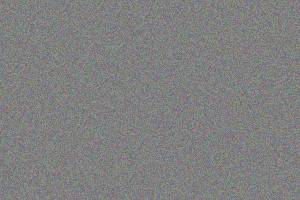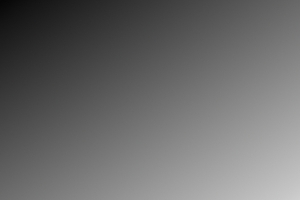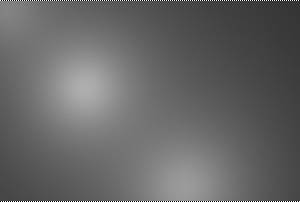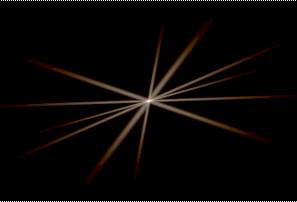## IceMan Reference Guide

#### Creation

Strictly speaking, these are not "operators" in the conventional sense, because they create new IceImage  instances.  They are accessed from the scripting language by sending the class name IceImage the messages below. This would be analogous to using a static member function in C++.

All these operations create a leaf node: a node with no inputs forming the leaves of an image processing expression.

Card(type, color)
type: Pixel data type (Number)
color: Pixel data as a float array (List)

Create a card, or an image of constant color. Cards are evaluated upon creation, unlike other images.

FilledImage(type, ply, box, color)
type: Pixel data type (Number)
ply: Number of channels (Number)
box: Image rectangle (List)
color: Pixel data as a float array (List)

Create an image of a specified type, ply and size that's filled with a specified color.  This is like a card, except that it has finite dimensions, and is not allocated until used.

GaussianNoise(type, ply, box, muAndSigma, range, seed)
type: Pixel data type (Number)
ply: Number of channels (Number)
box: Image rectangle (List)
muAndSigma: Mean and standard deviation (List)
range: Range of values in image (List)
seed: Large Number for random number generator seed (Number)

Create an image filled with Gaussian noise in the specified range. Mean and standard deviation are specified as a pair of floating point values.

PolygonFill(color, pointList, samples)
color: Card specifying fill color (Card)
pointList: Polygon vertex list (List of List)
samples: Super-sampling grid for anti-aliasing (List)

Create an image with a filled polygon. Edges are anti-aliased using a super-sampling grid specifed in samples. (5, 5) is usually sufficient.``` color := IceImage Card(IceComponentType Float, 1.0, 0.5, 0.3) vertices := list(list(228, 343), list(387, 218), list(478, 357), list(682, 233), list(746, 338), list(778, 450), list(629, 547), list(391, 545), list(388, 348), list(332, 331), list(275, 398), list(189, 386)) samples := list(5, 5) p := IceImage PolygonFill(color, vertices, samples) ```
UniformNoise(type, ply, box, range, seed)
type: Pixel data type (Number)
ply: Number of channels (Number)
box: Image rectangle (List)
range: Range of values in image (RealPoint)
seed: Large Number for random number generator seed (Number)

Create an image filled with values uniformly distributed in the specified range.``` size := list(0, 0, 300, 200) range := list(0.4, 0.6) seed := 398493 p := IceImage UniformNoise(IceComponentType Fractional, 3, size, range, seed) ```
Ramp(type, ply, box, angle, center, width, clampVal, easeInOut)
type: Pixel data type (Number)
ply: Number of channels (Number)
box: Image rectangle (List)
angle: Angle of ramp in degrees (Float)
center: Center of ramp (RealPoint)
width: Width of ramp (Float)
clampVal: Minimum and maximum values (RealPoint)
easeInOut: Ease-in and ease-out points (RealPoint)

This is a function to create a general color ramp.  The angle is specified in degrees from the vertical.  The center of the ramp is where the pixel value is exactly half-way between minimum and maximum values. The width is  specified in pixels along the direction of variation. The range of values in the ramp is restricted to clampVal. The parameter easeInOut contains the ease-in point, below which the ramp smoothly reduces to the minimum value, and the ease-out point, above which the ramp smoothly "decelerates" to the maximum value. Ease-in/out points are expressed as fractions of the total ramp width.

In the example shown below, the angle of the ramp is 32.3, its center is at pixel coordinates (150.6, 100.1), its width is 363.4 pixels, its values are clamped between 0 and 0.85, and the ease-in and ease-out points are 36.34 pixels from either end of the ramp.``` size := list(0, 0, 300, 200) center := list(156.2, 100.1) minAndMax := list(0, 0.85) ease := list(0.1, 0.1) p := IceImage Ramp(IceComponentType Float, 3, size, 32.3, center, 363.4, minAndMax, ease) ```
RBFInterp(dataBox, pointList, valueTable, rFrac, freezeEdges)
dataBox: Image rectangle
pointList: List of pixel coordinates (may be non-integral)
valueTable: Tabulated values of function at all pixel coordinates in pointList.
rFrac: A measure of the "sphere of influence" around a tabulated point. Useful values are in the 0.1 Ð 1 range: use smaller values for "tighter" roll-off.
freezeEdges: If true, edges are constrained to be zero valued.

Given the values of a function f(x, y) at arbitrary points on the 2D pixel planes, compute values for all points on the pixel grid by radial basis function interpolation.``` size := list(0, 0, 300, 200) centers := list(list(10, 10), list(50,50), list(200,100)) valuesAtCenters := list(0.4, 0.6, 0.8) sphereOfInfluence := .5 freezeEdges := 0 p := IceImage RBFInterp(size, centers, valuesAtCenters, sphereOfInfluence, freezeEdges) ```
Sparkle(sparkleType, imageSize, center, type, slitAngle)
sparkleType: Fraunhoferslits or GaussianSparkle, for sparkle and glow respectively. (Number)
imageSize: Size of image (not rectangle: origin assumed to be zero). (List)
center:  Center of sparkle. (List)
type:  Pixel data type for image. (Number)
slitAngle: Angle of slit for diffraction. (Number)

Sparkle and glow kernels for selective convolution. This operation produces 3-channel kernels for sparkle and glow effects. It is typically used in conjunction with ConvolveSelective and ConvolveTrig.``` sizePoint := list(300, 200) center := list(150,100) slitAngle := 23 kind := IceSparkleType FraunhoferSlits p := IceImage Sparkle(kind, sizePoint, center, IceComponentType Fractional, slitAngle) ```